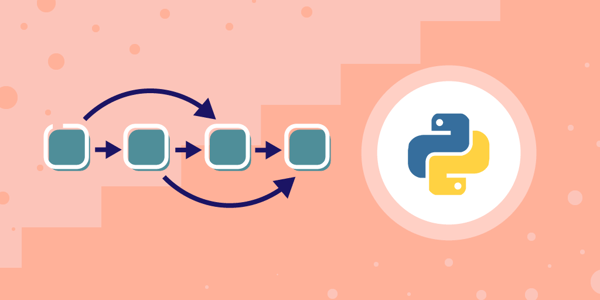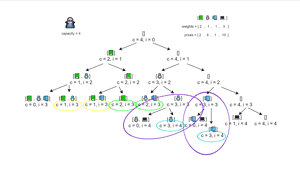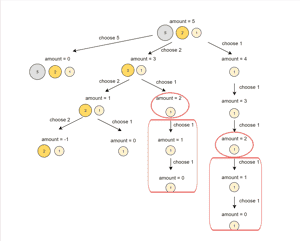# Dynamic Programming Tutorial: making efficient programs in Python

Jul 30, 2020 - 12 min readRyan ThelinIf you’re learning Python, it can be hard to take that step from writing sample code to efficient code. As you progress in skill, program runtime efficiency becomes more and more important, acting as a key indicator of success in coding interviews and later real-world solutions.

One way to get more efficiency out of your recursive programs is to start using dynamic programming, a time-saving storage-based technique, in place of brute force recursion. Dynamic programming uses the principle of optimality, which is the idea that if all steps of a process are optimized, then the result is also optimized. In our case, the optimal step is the one which takes the least amount of time which in programming means it takes the fewest new computations.

To help you jump into efficient Python code, here’s a quick tutorial on what dynamic programming is, why it’s more efficient, and how to use it to solve common interview problems.

## What is Dynamic Programming?

Dynamic programming is a problem-solving technique for resolving complex problems by recursively breaking them up into sub-problems, which are then each solved individually. Dynamic programming optimizes recursive programming and saves us the time of re-computing inputs later.

This differs from the Divide and Conquer technique in that sub-problems in dynamic programming solutions are overlapping, so some of the same identical steps needed to solve one sub-problem are also needed for other sub-problems.

This leads us to the main advantage of dynamic programming. Instead of recomputing these shared steps, dynamic programming allows us to simply store the results of each step the first time and reuse it each subsequent time.

Note: Dynamic programming is a special case of the larger category of recursive programming, however not all recursive cases can use dynamic programming

### Why is Dynamic Programming efficient? Recursion vs. DP

If the two are so closely entwined, why is dynamic programming favored whenever possible? This is because brute force recursive programs often repeat work when faced with overlapping steps, spending unneeded time and resources in the process.

Dynamic programming solves this issue by ensuring each identical step is only completed once, storing that step’s results in a collector such as a hash table or an array to call whenever it’s needed again. In doing so, dynamic programming allows for less repeated work and therefore better runtime efficiency.

Note: Dynamic programming essentially trades space efficiency for time efficiency as solution storage requires space not used in brute force recursive solutions.

### Dynamic Programming Examples

#### Real-world Dynamic Programing

Unlike computers, memory-based processes like dynamic programming are intuitive to humans. Consider this real-world example:

Imagine you want to go to a new grocery store a few streets away, but you don’t know how to get there. To solve the sub-problem of the route to the store, you look up directions on your smartphone’s map and then make your way there.

If you thought in a recursive fashion, each time you wanted to go to the store, you’d have to take the time to look up the directions again.

Instead, we naturally think dynamically, remembering the directions to the store from when we first looked them up, and as a result we don’t need to spend the time looking them up when we go in the future.

This is in essence how dynamic programming functions in programs as well.

#### Factorial Problem

We can see in real life how dynamic programming is more efficient than recursion, but let’s see it in action with Python code!

Below we have two solutions that both find the Fibonacci number of a given input and then show a graph of the program’s runtime. The left tab is simple brute force recursion, and the right instead uses dynamic programming.

Look at the difference.

import time
import matplotlib.pyplot as plt

def fib(n):
if n <= 0: # base case 1
return 0
if n <= 1: # base case 2
return 1
else: # recursive step
return fib(n-1) + fib(n-2)

numbers = 20
Recursion vs Dynamic
Recursive Time Complexity
1 of 2

As you can see, in the code of the dynamic solution we’ve added the ability to store old results (line 12) before moving onto the next recursive step (lines 13 - 15). We’ll see more complex dynamic solutions and their step-by-step breakdowns later in the article.

Notice when comparing each graph how the near-linear time complexity, $O(n)$, of the dynamic solution makes it perform vastly better even at later numbers when compared to the recursive function’s quadratic time complexity of $O(2^n)$.

## Bottom-Up Dynamic Programming

Not all dynamic solutions work in the same way. Some are built bottom-up while others are built top-down. The distinction can be found in how each begins a problem and how sub-problem results are stored.

Bottom-up dynamic programming solutions start by looking at the smallest possible sub-problem, called the base case, and then works step-by-step up to each sub-problem. As each sub-problem is solved, its solution is saved and used to solve the next lowest sub-problem. In the end, these building solutions will lead to an answer to the main problem.

### What is Tabulation?

Tabulation is the process of storing results of sub-problems from a bottom-up approach sequentially.

In tabulation, we don’t pick and choose which sub-problems need to be solved and instead solve every sub-problem between the base case and the main problem. This sequential order lends tabulation to use either lists or array, as those collections organize information in a specific order.

Note: Tabulation is iterative, not recursive, as in tabulation we complete each sub-problem before beginning the next.

## Top-down Dynamic Programming

Top-down dynamic programming is the opposite to bottom-up. A top-down solution first looks at the main problem and breaks it into smaller and smaller necessary sup-problems until the base case is reached. This is the most common way of building recursive solutions.

In using this style of recursive chain, top-down dynamic programming only solves sub-problems as they are needed rather than solving all in order.

### What is Memoization?

Memoization is the process of storing sub-problem results in a top-down approach.

Since top-down approaches solve problems as needed, memoization must store data in a non-sequential way. This means hashtables are the best collection type, as they store data in an unordered way.

In Python, this is best accomplished using the dictionary data structure because we can use it to store unordered data with key/value pairs.

## Dynamic Programming Examples: Bottom-up vs Top-down

To better understand the differences between these designs, let’s see how we’d rework our Fibonacci example in both a bottom-up and top-down way.

def fib(n):
if n == 0:
return 0
if n == 1:
return 1
# table for tabulation
table = [None] * (n+1)
table = 0        # base case 1, fib(0) = 0
table = 1        # base case 2, fib(1) = 1
# filling up tabulation table starting from 2 and going upto n
for i in range(2,n+1):
# we have result of i-1 and i-2 available because these had been evaluated already
table[i] = table[i-1] + table[i-2]
# return the value of n in tabulation table
return table[n]

print(fib(100))

## Practice Dynamic Programming Problems

Now give it a try yourself! Here’s some practice questions pulled from our interactive Dynamic Programming in Python course. Many of these problems are common in coding interviews to test your dynamic programming skills. Having a familiarity with these problems will make you a better candidate.

Try to figure out if each problem is best solved with bottom-up or top-down. If you get stuck, feel free to check the hints or solution.

### Knapsack Problem

Problem Statement:

Given a list of weights and a list of costs, find the optimal subset of things that form the highest cumulative price bounded by the capacity of the knapsack.

You should not change the signature of the given function; however, you may create a new function with a different signature and call it from the provided function.

Try to think of a simple solution to this problem. Once you have implemented that, add the memoization to account for more complex problems.

Sample Inputs:

weights = [1, 2, 4, 6]

prices = [4, 2, 4, 7]

capacity = 7

def knapsack(weights, prices, capacity):

return 0

Solution Breakdown:

In this problem, it is a little hard to see overlapping problems, since they do not follow a specific pattern. But if you look closely, you will notice capacity and index tuple are oftentimes repeating. All of these sub-problems have the same answers. Look at the following visualization.As we can see from the visualization, we can memoize based on a tuple of capacity and index. That is the only add-on in this code over the previous one with simple recursion. Before the recursive step, we check if the result is already available to us in the memo table. Similarly, after the evaluation of the result, we store it in the memo table again.

### Coin-Change Problem

This is a version of the “Coin-Change Problem” commonly asked in coding interviews.

Problem Statement:

Given a list of currency bills, you are required to count the number of ways in which you can represent a certain amount. For example, if you have only two kinds of bills, $10 and$20, and you want to represent $30, there are only two ways: • 3 bills of$10

• 1 bill of $20 and 1 bill of$10.

As you design the algorithm, take special care that you do not overcount. $30 can be represented with$20+$10 as well as$10+$20, but these are the same thing. Therefore, you should not count both cases. A simple recursive solution will timeout for large inputs; thus, you should try to write a dynamic programming algorithm. Start with a recursive solution and build up to a dynamic solution. def knapsack(weights, prices, capacity): # your code goes here return 0 Recursive Solution Breakdown: Finding different combinations in a set of things is not difficult. In fact, one of the first problems we did in this course was finding different permutations of a string. The challenging bit was how to avoid overcounting due to the same permutations. For example,$10 + $20 adds to$30, so does $20 +$10. In the context of permutations, both of these sequences would be distinct, but in the case of combinations, these are the same, meaning we must not count them twice. We achieve this by restricting each recursive call to use a subset of bills.

The key part of this solution is the sum of two recursive calls in line 7. To count to amount, some combinations will either include a specific bill given by bills[index] or they won’t. So, we can simply count both these possibilities. The first call to countways_ counts bills[index] as a part of the solution, whereas the second call skips over it. Let’s see a simple visualization of this algorithm.

# dynamic solution
def countways(bills, amount):

return 0

Dynamic Solution Breakdown:

This implementation is based on the algorithm we discussed in solution one.

To make up an amount using n bills, we just need to count the ways that we can either make the amount using the nth ​​ bill (lines 8-9) or without using it (lines 12-13). The algorithm is easier to understand with the visualization given below.For more dynamic programming problems, check out 6 Dynamic Programming problems for your next coding interview.

## What to learn next

After completing our tutorial, you can hopefully see how powerful a tool this is for Python developers. Not only are these concepts tested in coding interviews but they’re also essential for creating efficient real world Python applications.

Now that you’ve taken your first steps, the best thing to do is study up on when to use top-down vs bottom-up and to keep practicing more problems of various types.

Educative’s course Dynamic Programming in Python: Optimizing Programs for Efficiency is a great place to get all that you need to continue your journey. Within you’ll find dozens of lessons, deep-dives and practice problems, all written by Python developers to help you get hands-on experience.

By the end, you’ll know how to identify a dynamic programming problem, learned when to use top-down or bottom-up, and have enough in-depth practice to wow any interviewer.

## Wrapping up and resources

Congratulations on taking yet another step in perfecting your Python abilities. Today, we brought you through the what, why and how of dynamic programming and got some real interview question practice under your belt.

Moving from writing basic code to efficient code marks a great milestone in your learning. While it can be a steep learning curve, know that with each dynamic solution you create makes you that much more qualified and in-demand in our modern development space!